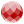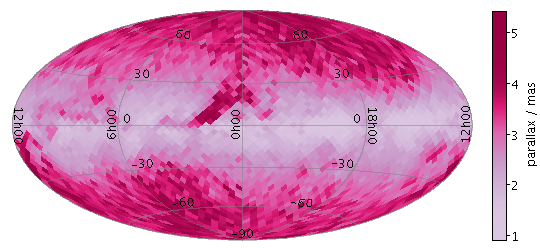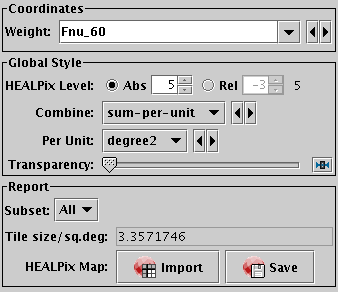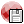Next Previous Up Contents
Next: Fill Form
Up: Plot Forms
Previous: Grid Form

#### A.4.5.19 SkyDensity Form

The SkyDensity form () plots a density map on the sky using a HEALPix grid with a configurable resolution. You can optionally use a weighting for the data values to accumulate within each HEALPix tile, and you can configure how the weighted values are combined to generate the eventual pixel values (and hence colours). HEALPix is a tiling scheme for the sky which uses square-ish pixels of equal area to cover the celestial sphere.

The shading is done using the shared colour map. This colour map is used by all currently visible SkyDensity, Grid, Aux and Weighted layers. When at least one such layer is being plotted, the Aux Axis control is visible in the control panel, which allows you to configure the colour map, range, ramp display etc.Example SkyDensity plotSkyDensity form configuration panel

The configuration options are:

Weight
The weight value applied to each plotted point. Fill this in with a column name or expression from the table just like for a positional coordinate. The exact way this quantity is used depends on the setting of the Combine control below. If it's left blank, the weighting is considered to be unity (all values are 1); this makes sense for some combination types (e.g. sum) but not others (e.g. mean).
HEALPix Level
This allows you to control the resolution of the HEALPix grid onto which the weighted values are resampled. According to the radio buttons, you can configure this using either a Absolute or Relative value. In Absolute mode you specify the HEALPix level (k) directly; the number of pixels on the sky is 12*22k. In Relative mode the level is set so that a HEALPix tile has approximately the given number of screen pixels along a side, and hence the absolute level will change if you zoom in and out. In either case, you can see the absolute level at the right hand side of the control.
Combine
Determines how the weight values associated with markers plotted covering a given HEALPix tile are combined to produce the numeric value used for that tile's colour.

For density-like values (`count-per-unit`, `sum-per-unit`) the scaling is additionally influenced by the Per Unit option.

The following options (some are more useful than others) are currently available:

• `sum`: the sum of all the combined values per bin
• `sum-per-unit`: the sum of all the combined values per unit of bin size
• `count`: the number of non-blank values per bin (weight is ignored)
• `count-per-unit`: the number of non-blank values per unit of bin size (weight is ignored)
• `mean`: the mean of the combined values
• `median`: the median of the combined values (may be slow)
• `q1`: the first quartile of the combined values (may be slow)
• `q3`: the third quartile of the combined values (may be slow)
• `min`: the minimum of all the combined values
• `max`: the maximum of all the combined values
• `stdev`: the sample standard deviation of the combined values
• `hit`: 1 if any values present, NaN otherwise (weight is ignored)

Per Unit
Defines the unit of sky area used for scaling density-like Combine values (e.g. `count-per-unit` or `sum-per-unit`). If the Combination mode is calculating values per unit area, this configures the area scale in question. For non-density-like combination modes (e.g. `sum` or `mean`) it has no effect.

The available options are:

• `steradian`: steradian
• `degree2`: square degree
• `arcmin2`: square arcminute
• `arcsec2`: square arcsecond
• `mas2`: square milliarcsec
• `uas2`: square microarcsec

Opaque Limit
Determines transparency of the points. By default, they are fully opaque, but if you slide the slider to the right, they will become progressively more transparent.

The Report panel provides information calculated by the plot:

HEALPix Level
Reports the actual HEALPix level of the plotted tiles. This is not necessarily the value selected in the style configuration controls, since if tiles would be smaller than screen pixels, the gridding is automatically degraded. This value is also reported to the right of the HEALPix Level configuration control.
Tile size/sq.deg
Reports the size of each plotted tile in square degrees. This is simply a function of the actual HEALPix level.
HEALPix Map
This allows you to export the healpix map that you can see in the plot in the form of a table. The table has a row for each plotted healpix pixel, with two columns: one giving the healpix pixel index, and the other giving the pixel value (corresponding to the colour in the plot). The Import () option loads the data as a new table in the TOPCAT application, and the Save () option lets you save it directly to disk in one of the available table formats. This information is also available from the Export menu.

Some notes apply for this export:

1. If you want to export the table following the HEALPix-FITS convention in a form suitable for use in other applications such as Aladin, you should save it using the output format fits-healpix rather than one of the other FITS variants.
2. When exported as FITS, the sky coordinate system (FITS `COORDSYS` header) is determined by the current value of the View Sky System, as reported in the Sky Axes Control.
3. The HEALPix level at which the data is exported is that currently plotted as reported by the HEALPix Level report item above, not necessarily the one selected in the style configuration controls.

Next Previous Up Contents
Next: Fill Form
Up: Plot Forms
Previous: Grid Form

TOPCAT - Tool for OPerations on Catalogues And Tables